Monsters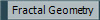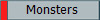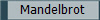Before fractal geometry was actually made into a kind of math we had been realizing that nature behaves differently than geometry wants it to. Mathematicians drew strange shapes that geometry could not explain. Listed are some of these shapes and people who studied mathematics that “broke the rules.”Cantor Dust This was the first of the monsters. Henry Smith discovered it in 1875, but Greog Cantor got it named after him. This was the first of the fractals. This “monster” took a line, cut it into three, and removed the middle third. Then it repeated on the two lines left over and the next four and so on. If you zoomed in on one of the small lines it would have the same branching pattern as the first.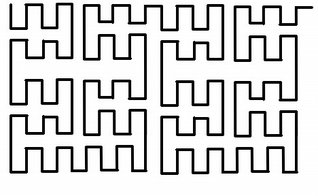The Peano Space It was discovered in Giuseppe Peano in 1890. This squiggly line was a counterexample to the statement: Lines don’t take up space. The Peano Space was a space-filling curve. It filled every requirement for a curve, but it did something unusual. It visited every point on a graph, meaning it took up space.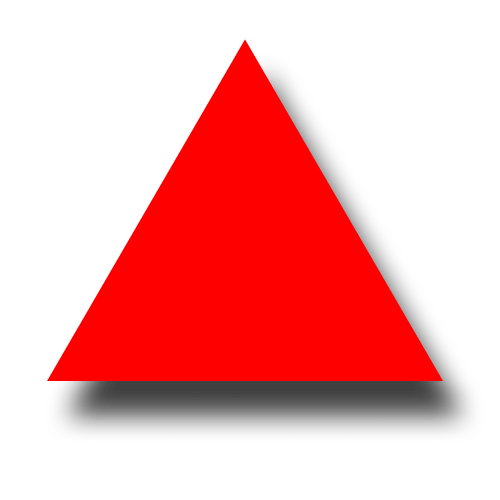Koch Curve/ Snowflake Helge von Koch discovered this monster in 1904. This fractal was also a curve. It took a line and put a triangle in the center. Then on each section it added another triangle, and then it repeated. This meant that the curve had an infinite measure. It could be infinitely long and is infinitely precise.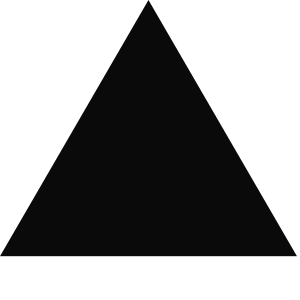The Sierpinski Gasket Vaclav Sierpinski discovered the Sierpinski Gasket in 1916. It took an equilateral triangle and drew an upside down triangle in the center. Then on the three smaller equilateral triangles the step repeated. If you zoomed in on the triangle it would exactly the same as the first part. A special property about the Sierpinski Gasket is that it could be made with the Pascal’s Triangle. If you shaded the odd numbers in on the triangle, the Sierpinski Gasket’s pattern appears.

Each of these “monsters” set the definition of fractal and got the ball rolling for fractal geometry. Mathematicians did not approve of these shapes. They were not smooth like most shapes they were used to. They considered these shapes just to be drawings, nothing mathematical.

## Gaston Julia

He studied the complex plane and how it works. Julia was interested in what happens when you take a number and put it into a formula, then take the number you get out and put it back in again. Julia made many different formulas, but he would never see what they looked like when graphed.

Poincaré

1884 the King of Sweden and Norway, Oscar II, asked a Swedish professor to organize a math competition. The goal was to prove that planets follow cycles. Poincaré participated in this competition. He proved that three planets will always follow a cycle, and he won the prize. However he realized that he made a mistake and that planets may not follow cycles. He then made formulas that created strange, seemingly unpredictable patterns. This is when "chaos" was found.This is an animation of a graph describing chaos.